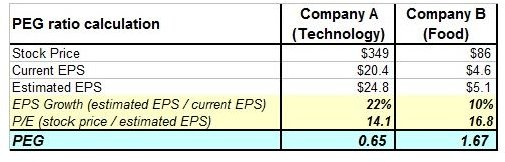# Understanding PEG Ratio in Stock Valuation

## Defining the PEG Ratio

The price/earnings to growth (PEG) ratio is used to compare a stock’s price/earnings (P/E) ratio to its expected annual earnings per share (EPS) growth rate. By using the P/E ratio — that actually compares a company's current share price to its earnings per share — the PEG ratio is an indication of a firm’s expected growth relatively to a stock’s price/earnings.

PEG ratio can be calculated as: PEG ratio = P/E Ratio / Annual EPS Growth

## Understanding PEG Ratio in Stock Valuation

Understanding PEG Ratio in stock valuation is of paramount importance. PEG ratio can be equal to one, greater than one or less than one. Depending on its value, the PEG ratio provides a relative valuation to how fairly priced, overvalued, or undervalued a stock is.

a) PEG ratio = 1

PEG ratio equal to 1 means that the stock is fairly priced to fully reflect its EPS growth. In an efficient market, the P/E ratio is expected to fully reflect a stock’s expected EPS growth.

b) PEG ratio > 1

PEG ratio greater than one means that the stock is overvalued or the market anticipates a higher EPS growth in the future. Typically, stocks with a PEG ratio greater than one are those for which the market expects higher growth in the future and investors are intrigued to undertake higher risk to receive higher returns.

However, earnings forecasts may be technically lowered. Automatically a lower EPS produces a higher PEG ratio as the EPS is the denominator in the formula used for the PEG ratio calculation. In this case, a PEG ratio greater than one does not denote a market expectation for a future higher growth, as, in spite of a higher PEG ratio, the stock price may be relatively stable.

c) PEG ratio < 1

The stock is undervalued or the market does not anticipate a higher EPS growth in the future. Typically, stocks with a PEG ratio less than one are those for which the market does not recognize a growth potential. On the other hand, a PEG ratio less than one may denote a technically higher EPS.

## Why the PEG Ratio is Useful?

To illustrate why the PEG ratio is useful, we assume that an investor is interested in buying stock in one of two different companies.

Company A is a technology company with a high growth potential.

Company B is a food company with a relatively stable growth potential.

One key factor to decide if the investment is valuable is the PEG ratio of each stock.

We assume that:The comparison of the two companies reveals that the growth of the technology stock does not justify its EPS. If no comparison was made, the investor would possibly buy the technology stock because most technology stocks are expected to have a high growth potential.

After the comparison, it seems that the technology stock is overvalued as its PEG ratio is lower than the PEG ratio of the food company, a stock that is not traditionally high growth.

The PEG ratio can assist investors to compare one company’s PEG to another and decide if investing in a particular stock is a valuable investment or the stock is overvalued.

## Are There Any Shortcomings in Using PEG?

Understanding PEG ratio and its use in stock valuation is easy. PEG can assist investors in effective investment decision-making because it considers both the P/E ratio and an expected growth rate. On the other hand, the PEG ratio is not always the best way to evaluate a potential investment.

The following are shortcomings in using PEG ratio.

1. Growth estimates are not always accurate as they may be based on analysts’ expectations and not on real growth potential. Moreover, estimates may change at any given moment.

2. Dividends are excluded from the PEG ratio calculation. In fact, the PEG ratio overlooks a company’s ability to increase shareholder value.

3. Inflation is not included in the PEG ratio calculation. If the growth of a company is the same as inflation, the PEG is a useless figure as, in fact, the company has no growth.

4. The PEG ratio is not really useful when assessing cyclical stocks, i.e. stocks that are closely related to the ups and downs of the economy. Typically, cyclical stocks demonstrate an increased demand when the economy grows and fall rapidly when economic growth is slowing down. As the earnings of cyclical companies tend to be extremely inconsistent, the PEG ratio is not practical.

5. Companies with low growth produce irrational PEG ratios that cannot be used for proper stock valuation.

6. Companies that have zero growth cannot be included in PEG calculations as dividing by zero is not possible.

In spite of its shortcomings, the PEG ratio is a reliable valuation method to identify undervalued, overvalued or fairly priced stocks. Generally, the lower the PEG, the better the value of a stock as investors would be paying less per unit of earning growth. However, the PEG ratio should be used complementary with other valuation methods to achieve reasonable results and should not be less than one.

## Resources

Image Credit: – PEG calculation created for this article by author Christina Pomoni